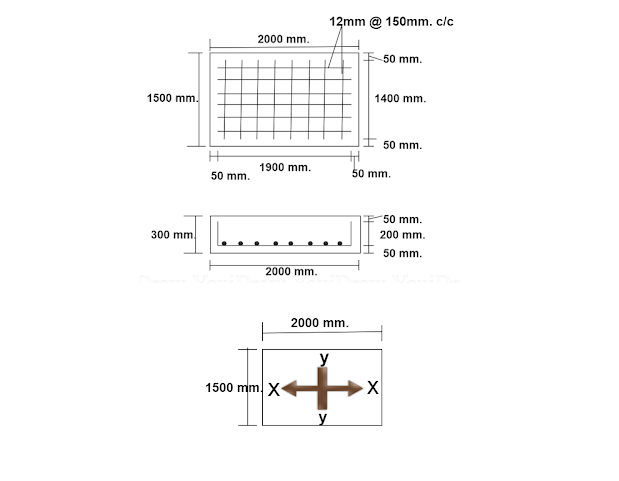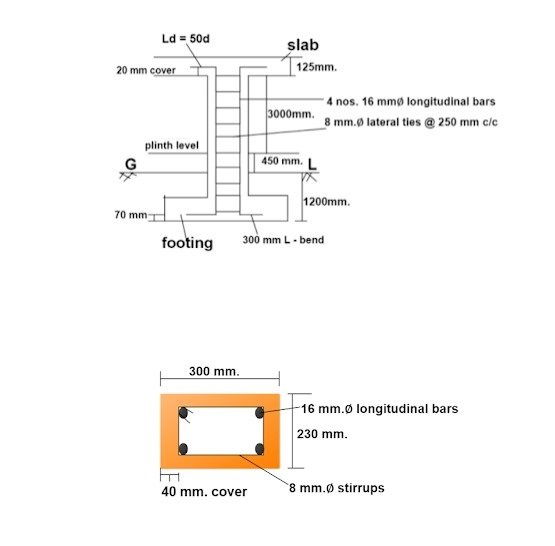Breaking News
Home / Civil Engineering / Bar Bending Schedule / Bar Bending Schedule Of Footing

# Bar Bending Schedule Of Footing

## Bar Bending Schedule Of Footing

Let us prepare the bar bending schedule of the footing for the below-given drawing.### Given data :

footing length = 2000 mm., width = 1500 mm. , depth = 300 mm.

rebar diameter = 12 mm.  spacing =150 mm, cover = 50 mm on all the sides.

No. of bars along x-direction

= [ {( footing length ) – ( 2 × cover )} ÷ spacing ] + 1

= [ {( 2000 mm.) – ( 2 × 50 mm.)} ÷ 150 mm.] +1

= [ { 1900 mm } ÷ 150 mm. ] +1

= 12.67 +1

### = 14 nos.

( by rounding off )

No. of bars along y-direction

= [ {( footing width ) – ( 2 × cover )} ÷ spacing ] + 1

= [ {( 1500 mm.) – ( 2 × 50 mm.)} ÷ 150 mm.] +1

= [ { 1400 mm } ÷ 150 mm. ] +1

= 9.33 +1

### = 10 nos.

Cutting length of the bar along the x-direction

=  [ {bar length in x – axis } +{ 2 nos. ×( L- bend length)}] – 2nos.× ( 2 times bar dia. for 90° bend.)

=[ { footing length – 2 × cover } + 2nos.×{ footing height – 2 × cover}] – 2× ( 2 × bar dia. )

= [ { 2000 mm. – 2 × 50 mm. } + 2 × { 300 mm. – 2 × 50 mm. } ] – 2 × ( 2 × 12 mm.)

= [ 1900 mm. + 400 mm ] – 48 mm.

= 2300 mm – 48 mm

= 2252 mm. i.e. 2.252 m.

Cutting length of the bar along the y -direction

=  [ {bar length in y – axis } +{ 2 nos. ×( L- bend length)}] – 2nos.× ( 2 times bar dia. for 90° bend.)

=[ { footing width – 2 × cover } + 2nos.×{ footing height – 2 × cover}] – 2× ( 2 × bar dia. )

= [ { 1500 mm. – 2 × 50 mm. } + 2 × { 300 mm. – 2 × 50 mm. } ] – 2 × ( 2 × 12 mm.)

= [ 1400 mm. + 400 mm ] – 48 mm.

= 1800 mm – 48 mm

= 1752 mm. i.e. 1.752 m.

## Bar Bending Schedule of the column drawing.## Given data :

Longitudinal bar dia. d = 16 mm.,  no. of bars = 4 no.

Lateral ties bar dia. d1= 8 mm., spacing = 250 mm. cover =40 mm.

Column size  x = 300mm  & y = 230 mm.

Development length Ld = 50d

Length of the longitudinal bar

= up to ground level + GL to plinth level + plinth level to slab bottom + slab cover + Ld + L- bend in footing – distance from footing bottom.

= {1200 mm.+ 450 mm. +3000 mm.+ 20 mm + 50d + 300 mm.} – 70 mm.

={ 4670 +( 50 × 16mm )+ 300 mm } – 70 mm.

= 5770 mm – 70 mm

= 5700 mm i.e. 5.70 m.

2. ### Bar Bending Schedule Of Plinth Beam

Length of the lateral ties

= perimeter of lateral ties + total hook length – no. of bends

= 2sides × ( x – 2 × cover ) + 2 sides × ( y – 2 × cover ) +( 2nos × hook length) – (3 nos. × bend )

( Here, we have taken  hook length = 10d1 for 135°∠    & bend = 2d1 for 90°∟)

={ [ 2 × (300mm – 2× 40mm.) ]+[ 2 × ( 230 mm – 2 × 40 mm.) ] } + { 2 × 10 × 8mm }- {3 × 2 × 8mm }

={ [ 2 × 220 mm ] + [2 × 150 mm ]} + 160 mm – 48 mm.

= {440 mm + 300 mm} + 112 mm

= 852 mm i.e. 0.852 m.

Total number of lateral ties ( stirrups )

={ [length of the longitudinal bar – (Ld + L bend over footing)] ÷ stirrup spacing } + 1

### Note:   Ld + L bend is deducted from the length as no stirrups are provided over that length.

={[ 5700 mm – (50 × 16 mm + 300 mm.)] ÷ 250 mm.} + 1

= {[ 5700mm – 1100mm ] ÷ 250 mm.} + 1

= {4600 mm ÷ 250 mm.} + 1

= 18.4 + 1

= 19.4 nos.

Rounding off, the number of stirrups required = 20 nos.

## Other Post

### THANKS.